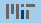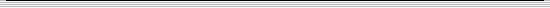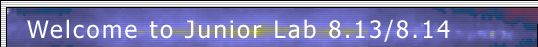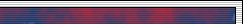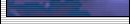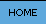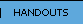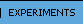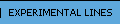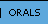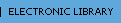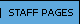Data AnalysisGetting Started with Matlab in Junior Lab2. A concise series of introductory lectures on MIT OCW explores basic MATLAB techniques
3. MIT also provides a web-based Matlab Tutorial (requires MIT certificates)
4. Matlab on Athena (Students can obtain a copy of Matlab here)

The Junior Lab Staff have provided a Matlab fitting template script to aid you in fitting the data you will acquire in Junior Lab. Download the following 6 files to a new directory on your local computer or within your Athena account.

1. fittemplate11.m (The main script)
2. fitlin.m (subroutine necessary for linear fitting functions)
3. levmar.m (subroutine necessary for non-linear fitting functions)
4. gradsearch.m (subroutine necessary for non-linear fitting functions)
5. gauss3.dat (a sample dataset used within the fitting template)
6. bev81.txt (a sample dataset used within the fitting template)
---Other---> NIST Statistical Data Sets

Matlab Examples for Inspiration
1. axisproperties.m Demonstrates various axis and grid options in figures

3. alphas.m Demonstrates the Geiger-Nuttall Relationship test in the Physics of Alpha Decay

4. subplots.m Demonstrates the use of the subplot command for efficient display of raw data sets

5. simpoisson.m Basic generation of Poisson distributed populations

6. singleslit.m Modeling single slit diffraction of a HeNe laser

7. doubleslit.m Models double slit diffraction

8. planck.m Calculates the spectral irradiance from a Black Body using the Planck Radiation Law

9. maxboltz.m Calculates the Maxwell-Boltzmann Distribution of Thermal Neutron Velocitites

10. expdecay.m Generates a noisy exponentially decaying dataset for testing non-linear fitting routines

11. xyerrorbars.m Demonstrates how to create plots with both x and y error bars

12. plotmultipleaxes.m Demonstrates how to create plots using both Y-axes (for data sets with very different scales)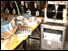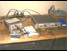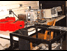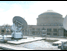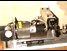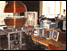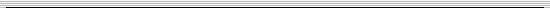site maintained by the Junior Lab Staff